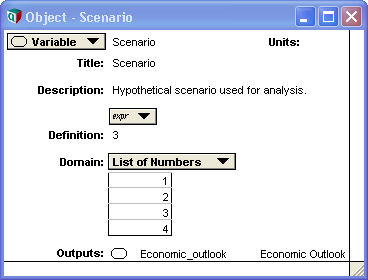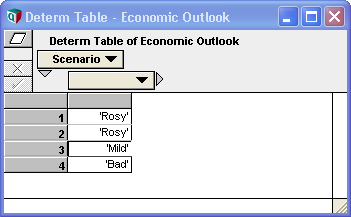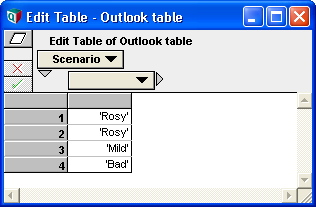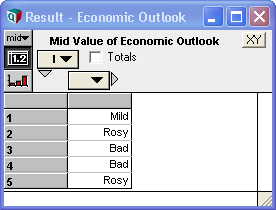# DetermTable

(Redirected from Determtable)

A DetermTable provides an input view like that of an edit table, allowing you to specify values or expressions in each cell for all index combinations; however, unlike a table, the evaluation of a determtable conditionally returns only selected values from the table. It is called a determtable because it acts as a deterministic function of one or more discrete-valued variables. You can conceptualize a determtable as a multi-dimensional generalization of a select-case statement found in many programming languages, or as a value that varies with the path down a decision tree.

To use a DetermTable, one or more of its table indexes should be discrete variables. These discrete variables must contain a domain attribute with a list of possible values. The domain can be either a List, List of Labels, or Index domain (in the case of an index domain, the possible values are taken from an index elsewhere in the model). These domain values serve as the index for the DetermTable's input view.

The value of the discrete variable specifies which slice of the DetermTable should be selected in the result. Only that row is returned. This can be contrasted to a normal edit table, in which the entire table (based on the discrete variable's domain) would be returned.

## Example

Here Scenario is a discrete variable. Its domain specifies the possible values, while its actual value is just one of those possible values:Because of the discrete domain, Scenario can serve as both an index and value (its IndexValue is [1, 2, 3, 4], while its value is 3). We can use it as an index for a DetermTable:When viewed in input mode, the DetermTable Economic Outlook appears as above, and you can change the values in each cell. When evaluated, only the row selected by Scenario is returned:## Creating a DetermTable

You can either create a DetermTable using the GUI or by typing it in as an expression.

To use the GUI, create a variable node and select Other... from the Definition type pulldown. If asked whether you intend to replace the definition, press OK. Then navigate to the Array library and select DetermTable. Press the Indexes button, which displays the index chooser, and select the index or indexes for your determtable. Then press OK twice to leave the wizards.

To type it as an expression, type:

DetermTable(I, J, K)

where I, J, and K are the indexes and discrete variables for your determtable. You may have between 1 and 15 discrete variables and indexes. After entering this definition, the definition converts to a DetermTable button, which you can press to fill in the cell values.

## Converting an existing Table to a DetermTable

The expression syntax for a DetermTable is identical to that of Table. Thus, one quick and easy way to convert an existing Table is to view the definition in expression view and change the first word from Table to DetermTable.

Before: Table(Scenario)('Rosy', 'Rosy', 'Mild', 'Bad')
After: DetermTable(Scenario)('Rosy', 'Rosy', 'Mild', 'Bad')

If you are converting a huge table (where the expression view contains more than 32000 characters), then Analytica will not allow you to edit the definition in expression view, so the above technique cannot be used.

A second method is to change the definition using the Object Finder. From the object window or attribute pane of the node currently defined as an edit table, view the definition attribute. Using the definition type pulldown, select "Other...". You'll be asked if you want to replace the current definition, which you do so press OK. The object finder appears, from you you should locate and select DetermTable from within the Array library. Press OK and your Table is converted to a DetermTable.

## Uses

### Discrete Probabilistic Models

DetermTables provide a natural mechanism for encoding utility (or cost) values in a discrete probabilistic model. This coordinates nicely with the use of ProbTables for encoding the uncertainties in discrete variables.

For an example of this use, see the Two Branch Party Problem.ana example model, included in the Example Models\Decision Analysis folder with the Analytica installation, where Value to author is defined as a DetermTable.

### Selective Parametric Analysis

When you want to evaluate your model for many different possible values on many different inputs, the very large parametric analysis that results may exceed available memory. Each time you convert an input value to a list of possible values, the computation and memory requirements increase multiplicatively. You may also have more than 15 inputs as potential parametric candidates, which exceeds the maximum dimensionality allowed by Analytica, even if you could fit it all in available memory.

To handle such large parametric analyses, you can utilize DetermTables to conduct Selective Parametric Analysis. You configure your model so that you can easily set only a subset of parametric inputs to lists -- the remainder are set to single values. After computing and viewing the result, then you can change to a different subset of parametric inputs. So for any given evaluation, you keep your dimensionality under control. For details on configuring a model in this fashion, see Selective Parametric Analysis.

## Subscript Equivalence

The functionality of a DetermTable can be implemented using two variables, the first containing a normal edit table, and the second containing a subscript function to select out the indicated row.

For example, to simulate the Economic Outlook DetermTable in the earlier example, we could use instead a normal Table with the values:We could then define Economic Outlook as:

Economic_outlook_table[Domain of Scenario = Scenario]

At first blush you might think the subscript operation is not doing anything. It looks a lot like re-indexing an array by replacing index Scenario with index Scenario. However, that is not the case. Remember that the discrete variable Scenario can serve as either an index or a value, depending on context. In a subscript operation, A[I = x], «I» is interpreted as an index, and «x» as a value. Hence, A[Scenario = Scenario] is saying to select the slice along the Scenario index specified by the value of Scenario. That is exactly what a DetermTable does.

Using a DetermTable has the convenience of saving you an extra node, since a separate node to perform the subscripting is not necessary. Perhaps more importantly, it is very easy to convert an existing table into a DetermTable without having to introduce a new node between the table and all its existing children.

If you ever need access to the full input table from your expressions (rather than just to the selected value), then you need to use a normal table with a subscript operator as just shown here.

Analytica 4.2 introduces one functional distinction between a determ table vs. the use of and edit table + subscript. In Analytica 4.2, the rows of a DetermTable that are not selected are not evaluated. This can be leveraged to save computation time, or continue with evaluation when unused cells result in errors.

Also new to Analytica 4.2, access to the full table for both a DetermTable and a ProbTable is possible using SubTable.

## DetermTable and Array Abstraction

DetermTables full array abstract when a decision variable contains additional dimensions. A very common example of this occurs when the decision variable is uncertain, so that in Sample mode, the probValue of the decision variable is indexed by Run. In this case, each Monte Carlo value separately selects its slice from the DetermTable.

In the Economic Outlook example above, suppose Scenario's definition was changed to:

Array(I, [3, 1, 4, 4, 2])

where Scenario's domain remains as before. Now Scenario's value is indexed by I. When the DetermTable in Economic_outlook is evaluated, array abstraction kicks in and selects the appropriate row separately for each value of Scenario, so that the evaluated result of Economic_outlook becomes:The decision variable can be self-indexed, as occurs when it is defined as Choice(Self,...) and All is selected. The values from the domain are the index values for the discrete value.

A variable in Analytica cannot have two separate index values. For a discrete variable, the domain provides the index values. Therefore, you cannot introduce a new list of values in the definition of a discrete variable. An attempt to define Scenario as the list [4, 1, 2] would result in an error, because the attempt to promote this unindexed list to a self-index would conflict with the presence of the domain attribute. If you want to introduce a list of values, you must create an array based on a separate index.

## Index domains for discrete variables

When creating a discrete variable, you may already have the list of possible values elsewhere in your model, in another index. There are several reasons why it may not make sense to re-enter these as a list or list-of-labels in the domain attribute. If you re-enter them, then if the set of values changes, you'll have to remember to change it in both places. It may be tedious to re-type or copy/paste the values. And if the set of values is computed, a list domain would not adapt if the computed list changes.

To use a the values from an existing index as the domain values for your discrete variable, select Index in the domain type pulldown when editing the domain attribute. After selecting, you are able to select the index that contains the possible values.

When using an index domain, your discrete variable functions as a totally separate index from your source index.

The index domain makes it easy to encode an arbitrary "switch" statement across any existing index. It solves the problem that your decision variable and your list of possibles values may not (initially) be the same object.